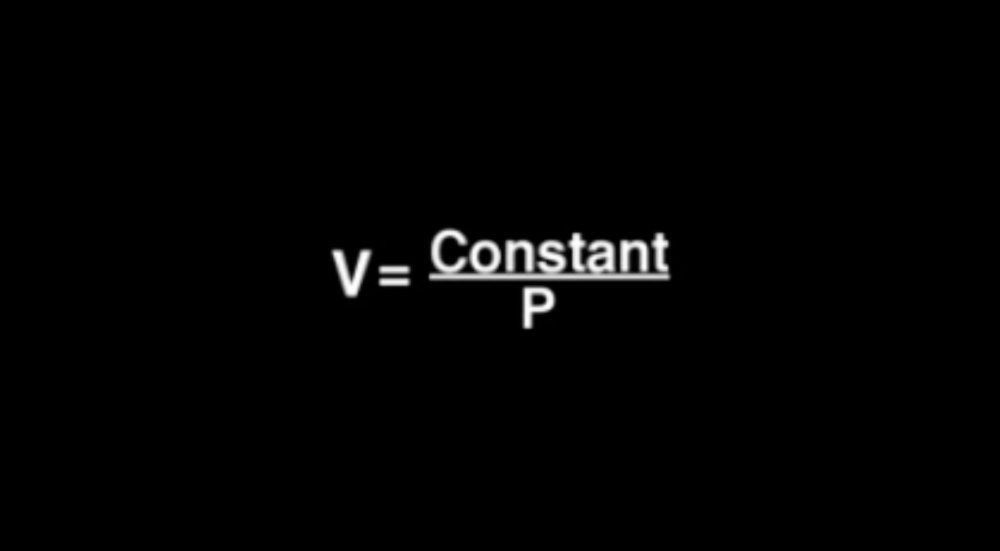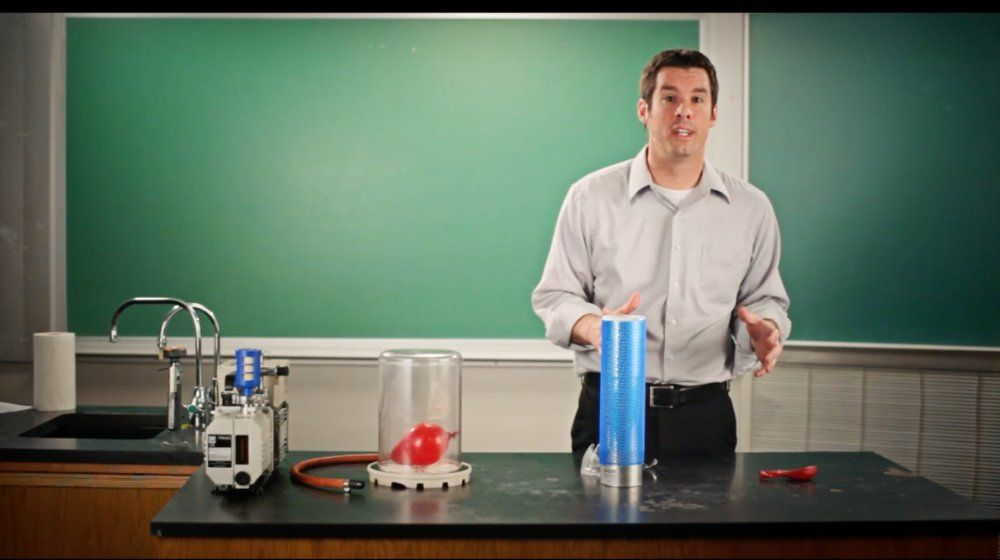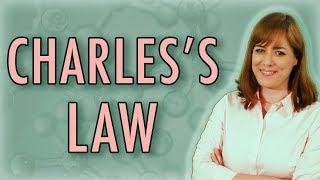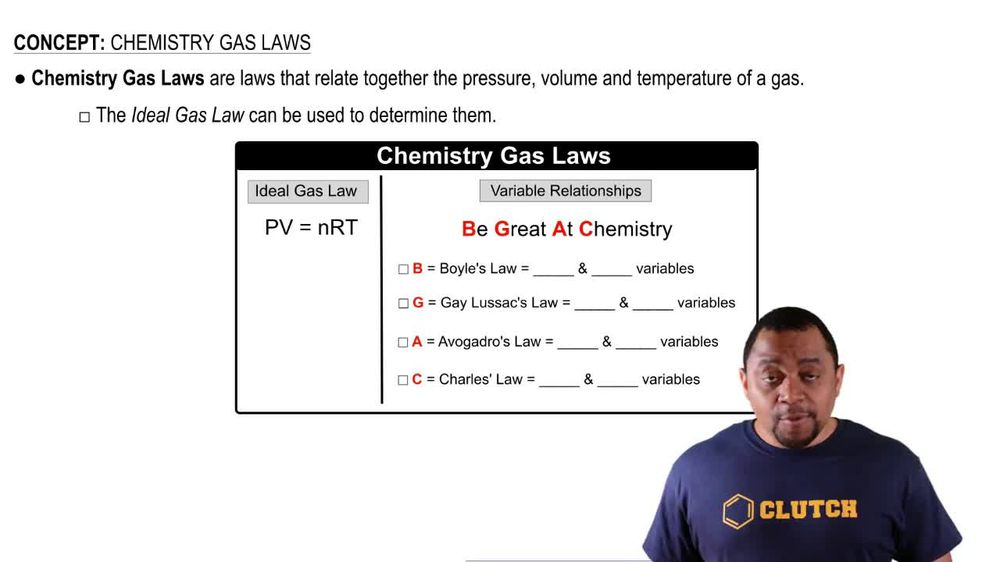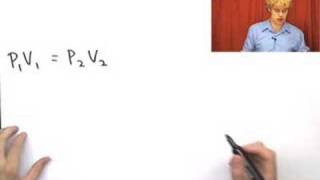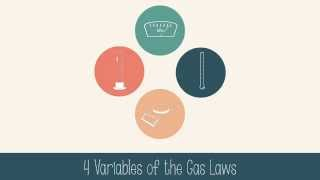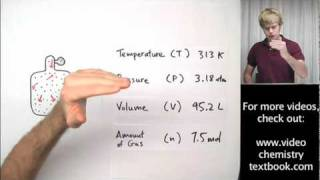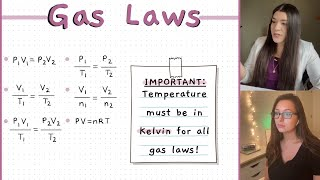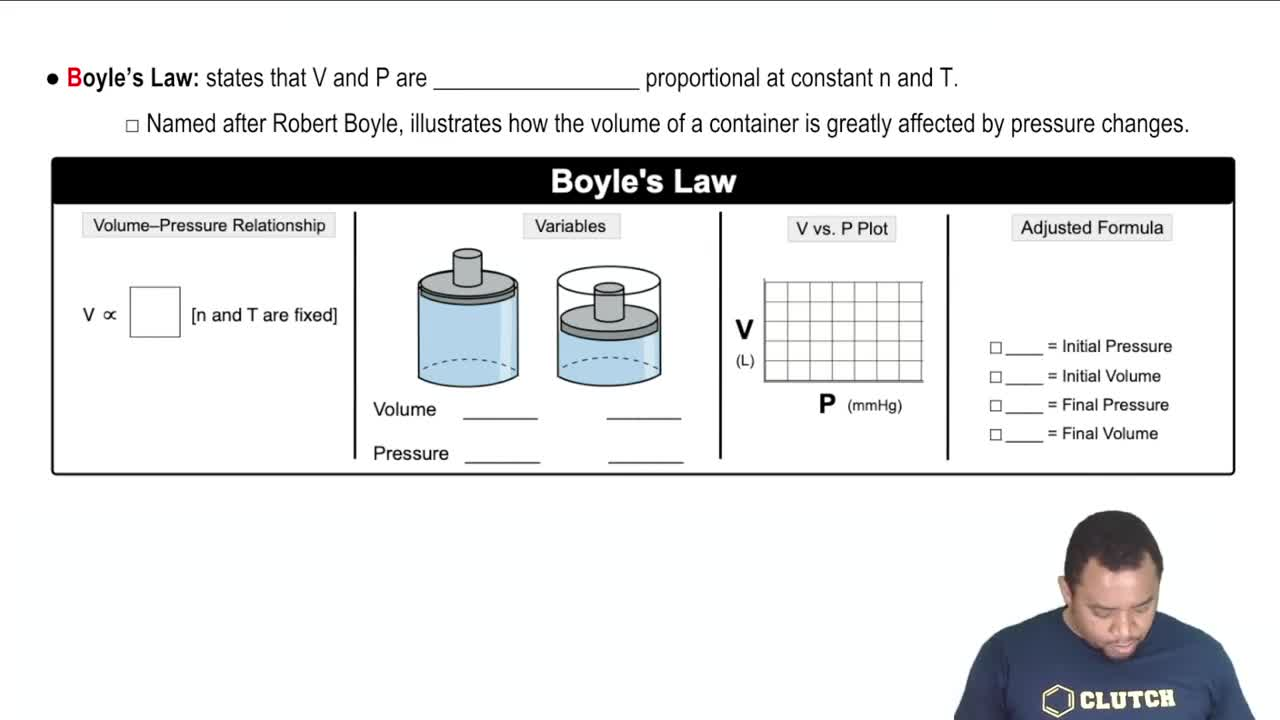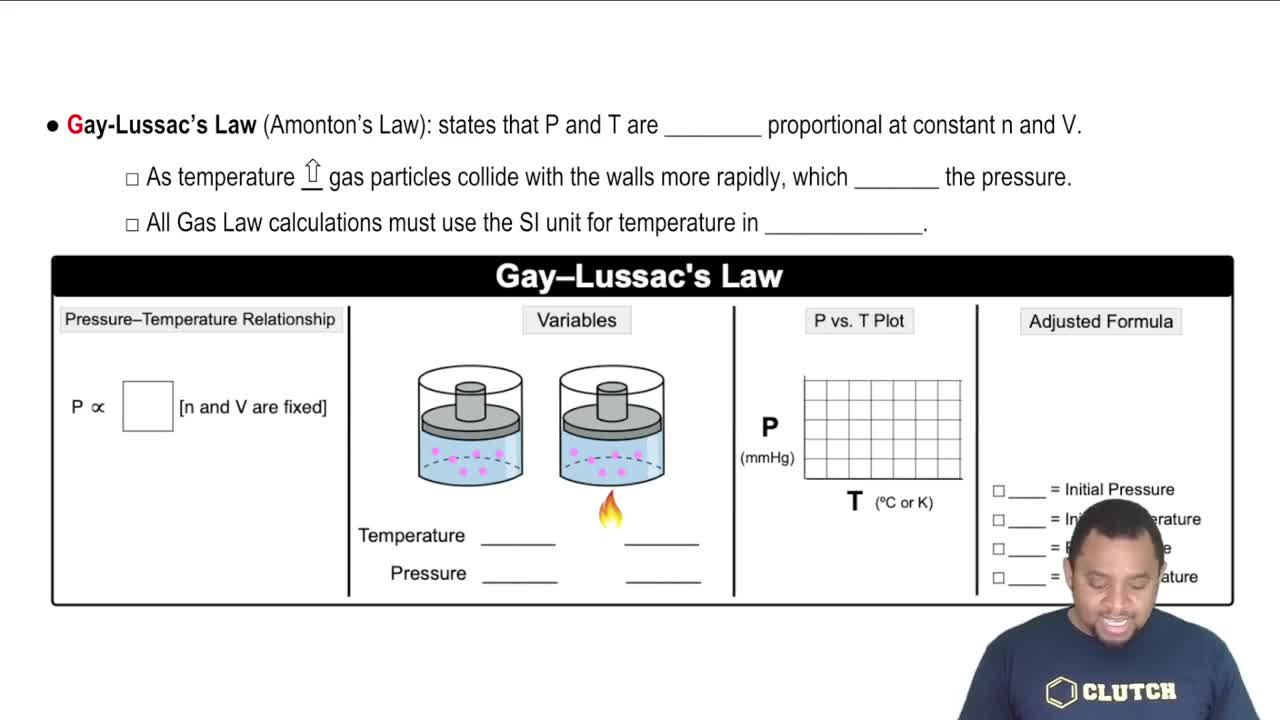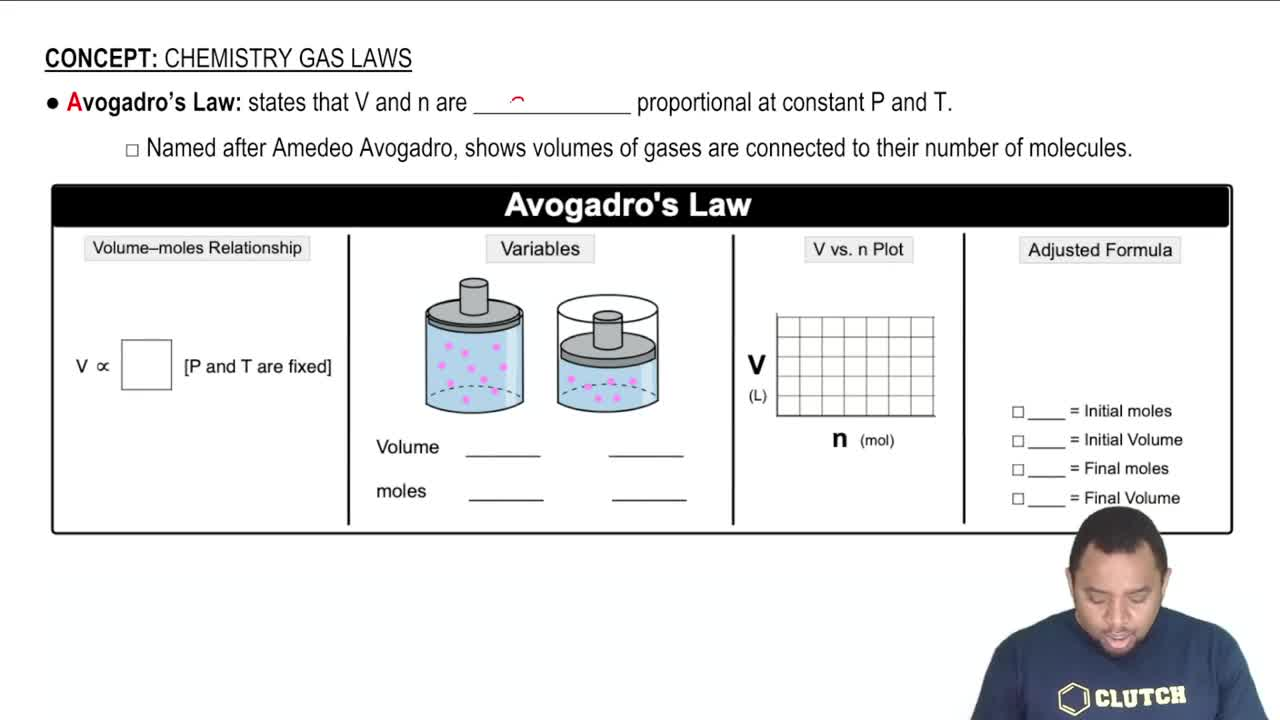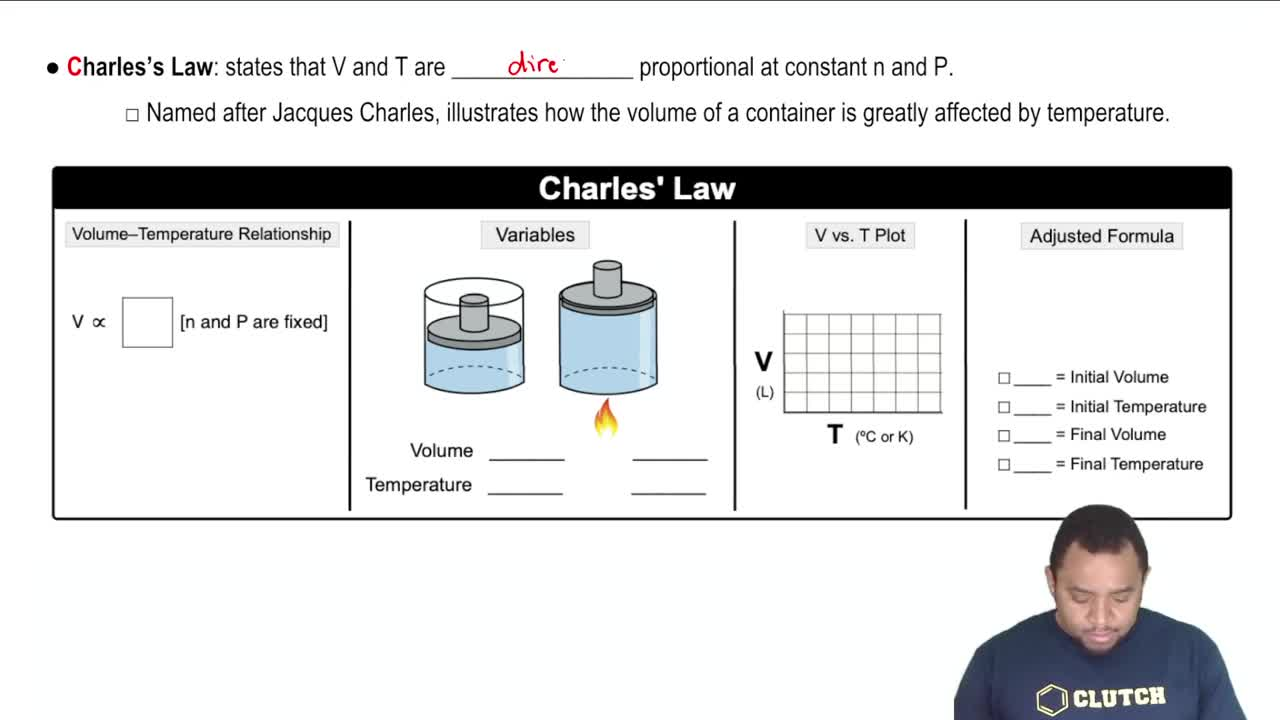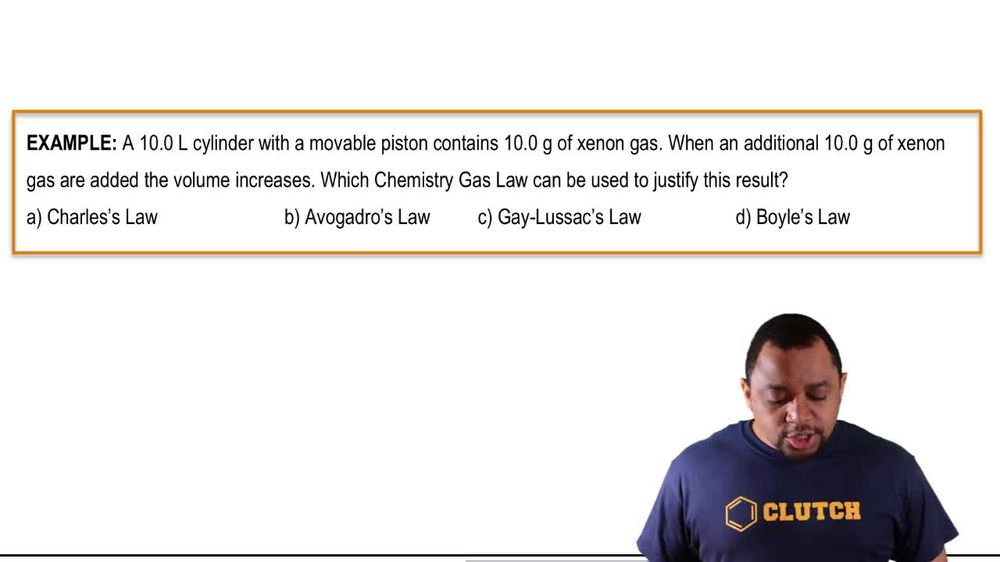Start typing, then use the up and down arrows to select an option from the list.
1. 7. Gases2. Chemistry Gas Laws# Chemistry Gas Laws

by Jules Bruno
99 views
1
1
now gay loose tax law, also known as amazon's Law says that pressure and temperature are directly proportional at constant moles and and volume. The Now as temperature increases our gas particles collide with the walls more rapidly, That's because they're absorbing the thermal energy and they're using it to propel themselves faster inside the container. And this will cause an increase in my pressure. Now remember pressure itself equals force over area. We said that the volume is constant, so your area would be constant, It's staying the same. If I'm increasing my temperature again, my gas will move faster inside the container. They're gonna hit the walls more rapidly, but also with more force. So my forces increasing my area is staying the same. This causes my pressure to increase. Okay, so that's why pressure and temperature are directly proportional. Now, we're going to say, remember that with all gas law calculations, we must use the S. I. Units for temperature in kelvin. So our units for temperature here are in kelvin. Now, what is the pressure temperature relationship there, directly proportional? So you just say that P is directly proportional to t and that happens when moles and volume are the same or fixed? Not the same, but fixed. How do we show this? Well, here we have two images of pistons, containers with pistons that are movable in the first image, I haven't applied a heat source. So we're going to say that our temperature here would be low, the temperature is low. So our molecules don't have extra outside energy to absorb. So they're not moving as vigorously as rapidly, They're not hitting the container with as much force and therefore a pressure would be low. But all of a sudden I had a flame. The container absorbs the heat, which eventually transitions to the molecules absorbing this heat, allowing them to move more rapidly and with greater force. So temperature is high, which eventually it's a greater force which leads to greater pressure. So pressure would be high. How would I depict this in a plot? They're both directly proportional. So we'd say that they both would be increasing together. So you'd have a line that's increasing over time. What would their adjusted Formula B or the gay loose sacks? Formula it would just be P one over T. One equals P two over T. Two. Again, take a look at my ideal gas law applications section on how we could derive this formula. Now we know that it's connected to the gay loose sacks or amazon's law. Now, remember with these variables, we'd say initial pressure is P one. Initial temperature is T one. Final pressure will be P. Two and final temperature would be T. Two. So remember when we're talking about gay loose tax law or amazon's law, that pressure and temperature are directly proportional when our moles and on our volume V. R. Constant or fixed